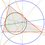# Double Counting on the Floor

Hey, Brilliant!

Today, I went to an AIME preparation day camp, and the instructor showed us a really neat proof of the following assertion:

$\lfloor \log_2 n \rfloor + \lfloor \log_3 n \rfloor + \lfloor \log_4 n \rfloor + \cdots + \lfloor \log_n n \rfloor = \lfloor \sqrt{n} \rfloor + \lfloor \sqrt{n} \rfloor + \lfloor \sqrt{n} \rfloor + \cdots + \lfloor \sqrt[n]{n} \rfloor,$

where $n$ is a positive integer greater than 1. If you want, you can stop reading right here and see if you can come up with a proof of the statement yourself. The proof I was shown begins after the line break below.

.

.

.

.

.

.'

.

.

.

.

.

.

OK, so here's the proof. First, let’s draw an $n \times n$ grid and label the columns 1 to $n$ from right to left, and the rows 1 to $n$ from top to bottom:

$\begin{array}{c|c|c|c|c|c} & 1 & 2 & 3 & \cdots & n \\ \hline 1 & & & & & \\ \hline 2 & & & & & \\ \hline 3 & & & & & \\ \hline \vdots & & & & & \\ \hline n & & & & & \\ \end{array}$

We fill the entry on the $x$th row and $y$th column with the number $x^y$:

$\begin{array}{c|c|c|c|c|c} & 1 & 2 & 3 & \cdots & n \\ \hline 1 & 1^1 & 1^2 & 1^3 & \cdots & 1^n \\ \hline 2 & 2^1 & 2^2 & 2^3 & \cdots & 2^n \\ \hline 3 & 3^1 & 3^2 & 3^3 & \cdots & 3^n \\ \hline \vdots & \vdots & \vdots & \vdots & \ddots & \vdots \\ \hline n & n^1 & n^2 & n^3 & \cdots & n^n \\ \end{array}$

Now, we will count the total number of entries in this grid that are less than or equal to $n.$ We will do this in two different ways: counting row by row, and counting column by column.

For the row by row method, let’s focus on a specific row $x.$ Observe that $x^{\log_xn} = n,$ so columns with value $\log_x n$ and below will produce entries that are less than or equal to $n.$ This gives us $\lfloor \log_x n \rfloor$ entries in the row that are less than or equal to $n.$ For row 1, all $n$ entries are clearly less than or equal to $n,$ so the total number of entries in the grid that are less than or equal to $n$ is $n + \displaystyle \sum_{i = 2}^n \lfloor \log_i n \rfloor.$

The column by column method works the same way. If we choose a specific column $y,$ then because $(n^{1/y})^y = n,$ all rows that are of value $n^{1/y}$ and below produce entries less than or equal to $n.$ This gives us $\lfloor \sqrt[y]{n} \rfloor$ total entries in column $y.$ Combined with the $n$ entries in column 1, the total number of entries in the grid that are less than or equal to $n$ is $n + \displaystyle \sum_{i = 2}^n \lfloor \sqrt[i]{n} \rfloor.$

Since both methods counted the same thing, we can equate the final expressions we got:

\begin{aligned} n + \displaystyle \sum_{i = 2}^n \lfloor \log_i n \rfloor &= n + \displaystyle \sum_{i = 2}^n \lfloor \sqrt[i]{n} \rfloor \\ \displaystyle \sum_{i = 2}^n \lfloor \log_i n \rfloor &= \displaystyle \sum_{i = 2}^n \lfloor \sqrt[i]{n} \rfloor, \end{aligned}

which is precisely the original claim. $\blacksquare$Note by Steven Yuan
3 years, 5 months ago

This discussion board is a place to discuss our Daily Challenges and the math and science related to those challenges. Explanations are more than just a solution — they should explain the steps and thinking strategies that you used to obtain the solution. Comments should further the discussion of math and science.

When posting on Brilliant:

• Use the emojis to react to an explanation, whether you're congratulating a job well done , or just really confused .
• Ask specific questions about the challenge or the steps in somebody's explanation. Well-posed questions can add a lot to the discussion, but posting "I don't understand!" doesn't help anyone.
• Try to contribute something new to the discussion, whether it is an extension, generalization or other idea related to the challenge.

MarkdownAppears as
*italics* or _italics_ italics
**bold** or __bold__ bold
- bulleted- list
• bulleted
• list
1. numbered2. list
1. numbered
2. list
Note: you must add a full line of space before and after lists for them to show up correctly
paragraph 1paragraph 2

paragraph 1

paragraph 2

[example link](https://brilliant.org)example link
> This is a quote
This is a quote
    # I indented these lines
# 4 spaces, and now they show
# up as a code block.

print "hello world"
# I indented these lines
# 4 spaces, and now they show
# up as a code block.

print "hello world"
MathAppears as
Remember to wrap math in $$ ... $$ or $ ... $ to ensure proper formatting.
2 \times 3 $2 \times 3$
2^{34} $2^{34}$
a_{i-1} $a_{i-1}$
\frac{2}{3} $\frac{2}{3}$
\sqrt{2} $\sqrt{2}$
\sum_{i=1}^3 $\sum_{i=1}^3$
\sin \theta $\sin \theta$
\boxed{123} $\boxed{123}$